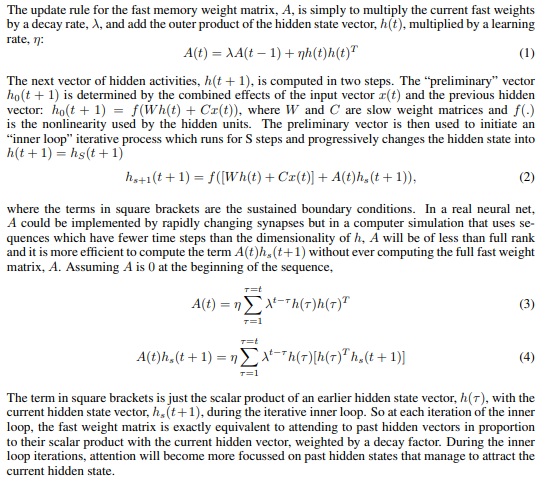# Inplace operation alternative to clone() (using too much memory)

I wrote a pytorch module to implement the fast weight RNN described in this paper:I use in place operations when I implement equation 4 in my code, and use clone() to make sure that my code works with autograd. The problem is that understandable, having clone() in a loop that runs for S steps incurs a significant memory penalty. I cannot run the code on my gpu as I only have 8gb and as it is now I am struggling to run even a training set of size 100 with 32GB RAM.

My question: Is there a better way to implement equation 4 from article?

I have attached my code:

Blockquote
#make fast RNN cell to be used in fastRNN

``````class FWRNNCell(nn.Module):
def __init__(self, input_size, hidden_size, fast_iter = 10, fast_decay_rate = .95, fast_learn_rate = .5):
super().__init__()
#initialize parameters
self.input_size = input_size
self.hidden_size = hidden_size
self.fast_iter = fast_iter
self.fast_decay_rate = fast_decay_rate
self.fast_learn_rate = fast_learn_rate

#initalize learnable input weight and weight matrix
self.C = nn.Parameter(torch.randn(self.hidden_size, self.input_size))#input weight matrix
self.W = nn.Parameter(torch.randn(self.hidden_size, self.hidden_size))#weight matrix

#initalize the learnable biases
self.bi = nn.Parameter(torch.randn(self.hidden_size,1))#bias input col vector
self.bw = nn.Parameter(torch.randn(self.hidden_size,1))#bias weights col vector

#instantiate the activation function function
#will be using tanh as this is default function for nn.RNN
self.tanh = nn.Tanh()

def forward(self, X, hidden):
#will only recieve one entry of the batch at once
#X will only have 2 dimensions
#some useful initializations
seq_len, input_size = X.size()
f_lr = self.fast_learn_rate
f_dr = self.fast_decay_rate

#attention check to see if input size is correct
#no need to check hidden as this will be called from method in fastRNN
assert  input_size == self.input_size, f'FWRNNCELL input_ size ({self.input_size}) not equal to input_size in input ({input_size})'

#initialize hidden list
h = torch.zeros(seq_len, self.hidden_size, 1)
h = torch.transpose(hidden.squeeze(0), 0, 1) #h must be a column vector

#initalize fast hidden list
f_h = [i for i in range(self.fast_iter+1)]
#get new hidden and new output
for t in range(1, seq_len):
#print('t: ', t)
#initialize
x_tmin1 = X[t-1].unsqueeze(1) #must be column vector

#initialize fw_h using hidden from previous
f_h = self.tanh((torch.matmul(self.W, h[t-1].clone()) + self.bw) + (torch.matmul(self.C, x_tmin1) + self.bi))
#the fast weight inner loop
#####################################
#IN PLACE OPERATIONS IN LOOP BELOW#
#####################################
for s in range(1, self.fast_iter+1):
#first part
a = (torch.matmul(self.W, h[t-1].clone()) + self.bw) + (torch.matmul(self.C, x_tmin1) + self.bi)
#second part
#initialize the first b tau = 0
b  = f_dr**(t-1)*torch.matmul(h.clone(), torch.matmul(torch.transpose(h.clone(),0,1), f_h[s-1].clone()))
for tau in range(t):#goes to t-1
b = b + f_dr**(t-1-tau)*torch.matmul(h[tau].clone(), torch.matmul(torch.transpose(h[tau].clone(),0,1), f_h[s-1].clone()))

#sum tanh(a+b)
#print('a size: ', a.size())
#print('b size: ', b.size())
f_h[s] = self.tanh(a + f_lr*(b))
#print('f_h size: ', f_h[s].size())
#insert layer normalization

#assign f_h[S] to h[t]
#print('assigning h[t]')
h[t] = f_h[-1]
#h[-1] is the hidden to pass to output
output = h.squeeze(2).unsqueeze(0)
hidden = output[-1].unsqueeze(0)

return output, hidden
``````

I would really appreciate any direct suggestions of things I can change in my code as well as references that could be of aid to me.

Note: I wrap my FWRNNCell in another module FWRNN which adds the ability to have multi-layer Fast weight RNN and also handles batches, but there are no in place operations performed in this class (apart from those that result from using FWRNNCell)

Thank you very much for your time,
Nico

Try changing h to tensor list, e.g.: h = list(h.unbind()). Then cloning shouldn’t be needed. Not sure why you do f_h[idx].clone(), f_h is a tensor list, so I don’t see inplace operations with its elements, just element replacements.

Inner (tau) loop looks optimizable/vectorizable. Matmuls done there may be redundant, i.e. could maybe be done (“cached”) in some place outside.

PS I didn’t delve too deep into semantics, these are just “mechanical” things.

Thank you! I made h a list and then used it to populate the output tensor. When I did this I no longer required the use of .clone() and that greatly reduced the amount of memory required.

I am having some trouble making it work on my gpu but I will figure that out tomorrow.

thanks again